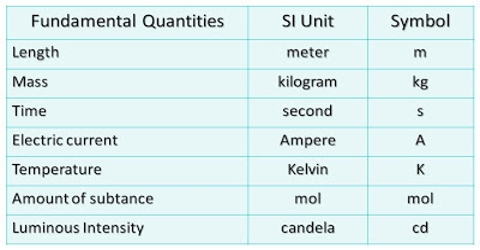# Fundamental and Derived Unit

Fundamental and Derived Unit

There is innumerable physical quantifies in physics each of which has its own unit. But almost all the units of physical quantities can be expressed in terms of three units. viz—

(1) Fundamental unit.

(2) Derived unit and

(3) Practical unit.

The unit that does not depend on any other unit and is totally independent is called fundamental unit.

For example, the unit of length or Mass or time does not depend on any other unit. So, the units of length, mass and time are fundamental units.

Depending on these units any other unit which is formed or derived from these fundamental units is called derived or compound unit. In other words, a unit formed based on these three units or a unit derived from fundamental units, is called a derived or compound unit. As for example, in order to measure area, we need to multiply length with breadth. If the unit of length as metre (m), then the area of a body of length of 1 metre and breadth of 1 metre = 1 metre (m) x 1 metre (m) = 1 metre2 or 1 m2.

This square metre (m2) is the unit of area. So, it is seen that if the unit of length is known, then unit of area can be known. Hence unit of area is a compound unit. Similarly, volume, velocity, force etc are compound unit.

There are three fundamental units: (a) Unit of length. (b) Unit of mass and (c) Unit of time.

These units will be fixed, convenient and unchangeable i.e., if the distance is 1 metre in summer, then that will be 1 metre in winter as well. It will not be changed with time or pressure etc.

Sometimes fundamental or primary units become very large or very small and hence is inconvenient for practical use. In those cases, their sub-multiples or multiples are used as units. Sometimes, some new units are also used. This is called practical unit. For example, kilometre (km), micron (μ), Ton etc. Of course, the question arises whether according to Einstein’s theory of relativity mass. time and length will be equal or same from all the places in the universe.